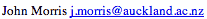Data Structures and Algorithms Graph Representations

#### Node Representations

Usually the first step in representing a graph is to map the nodes to a set of contiguous integers. (0,|V|-1) is the most convenient in C programs - other, more flexible, languages allow you greater choice! The mapping can be performed using any type of search structure: binary trees, m-way trees, hash tables, etc.

#### Adjacency Matrix

Having mapped the vertices to integers, one simple representation for the graph uses an adjacency matrix. Using a |V| x |V| matrix of booleans, we set aij = true if an edge connects i and j. Edges can be undirected, in which case if aij = true, then aji = true also, or directed, in which aij != aji, unless there are two edges, one in either direction, between i and j. The diagonal elements, aii, may be either ignored or, in cases such as state machines, where the presence or absence of a connection from a node to itself is relevant, set to true or false as required.

When space is a problem, bit maps can be used for the adjacency matrix. In this case, an ADT for the adjacency matrix improves the clarity of your code immensely by hiding the bit twiddling that this space saving requires! In undirected graphs, only one half of the matrix needs to be stored, but you will need to calculate the element addresses explicitly yourself. Again an ADT can hide this complexity from a user! If the graph is dense, ie most of the nodes are connected by edges, then the O(|V|2) cost of initialising an adjacency matrix is matched by the cost of inputting and setting the edges. However, if the graph is sparse, ie |E| is closer to |V|, then an adjacency list representation may be more efficient.

#### Adjacency List Representation

Adjacency lists are lists of nodes that are connected to a given node. For each node, a linked list of nodes connected to it can be set up. Adding an edge to a graph will generate two entries in adjacency lists - one in the lists for each of its extremities.

#### Depth-first Traversal

A depth-first traverse of a graph uses an additional array to flag nodes that it has visited already.

Using the adjacency matrix structure:

```struct t_graph {
int n_nodes;
graph_node *nodes;
int *visited;
adj_matrix am;
}

static int search_index = 0;

void search( graph g ) {
int k;
for(k=0;k<g->n_nodes;k++) g->visited[k] = FALSE;
search_index = 0;
for(k=0;k<g->n_nodes;k++) {
if ( !g->visited[k] ) visit( g, k );
}
}
```
The visit function is called recursively:
```void visit( graph g, int k ) {
int j;
g->visited[k] = ++search_index;
for(j=0;j<g->n_nodes;j++) {
if ( adjacent( g->am, k, j ) ) {
if ( !g->visited[j] ) visit( g, j );
}
```
This procedure checks each of the |V|2 entries of the adjacency matrix, so is clearly O(|V|2).

Using an adjacency list representation, the visit function changes slightly:

```struct t_graph {
int n_nodes;
graph_node *nodes;
AdjListNode *adj_list;
int *visited;
adj_matrix am;
}

void search( graph g ) {
... /* As adjacency matrix version */
}

void visit( graph g, int k ) {
AdjListNode al_node;
g->visited[k] = ++search_index;
al_node = ListHead( g->adj_list[k] );
while( n != NULL ) {
j = ANodeIndex( ListItem( al_node ) );
if ( !g->visited[j] ) visit( g, j );
al_node = ListNext( al_node );
}
}
```
Note that I've assumed the existence of a List ADT with methods,
• ListHead,
• ListItem,
• ListNext
and also a AdjListNode ADT with a
• ANodeIndex
method.

The complexity of this traversal can be readily seen to be O(|V|+|E|), because it sets visited for each node and then visits each edge twice (each edge appears in two adjacency lists).

#### Breadth-first Traversal

To scan a graph breadth-first, we use a FIFO queue.
```static queue q;
void search( graph g ) {
q = ConsQueue( g->n_nodes );
for(k=0;k<g->n_nodes;k++) g->visited[k] = 0;
search_index = 0;
for(k=0;k<g->n_nodes;k++) {
if ( !g->visited[k] ) visit( g, k );
}

void visit( graph g, int k ) {
al_node al_node;
int j;
AddIntToQueue( q, k );
while( !Empty( q ) ) {
k = QueueHead( q );
g->visited[k] = ++search_index;
al_node = ListHead( g->adj_list[k] );
while( al_node != NULL ) {
j = ANodeIndex(al_node);
if ( !g->visited[j] ) {
AddIntToQueue( g, j );
g->visited[j] = -1; /* C hack, 0 = false! */
al_node = ListNext( al_node );
}
}
}
}
```

### Key terms

Adjacency Matrix
A structure for representing a graph in which the presence of arcs between nodes is indicated by an entry in a matrix.
Adjacency Lists
An alternative structure for representing a graph in which the arcs are stored as lists of connections between nodes.
Breadth-first Traversal
Traversing a graph by visiting all the nodes attached directly to a starting node first.
Depth-first Traversal
Traversing a graph by visiting all the nodes attached to a node attached to a starting node before visiting a second node attached to the starting node.

 Continue on to Dijkstra's Algorithm Back to the Table of Contents
©, 1998